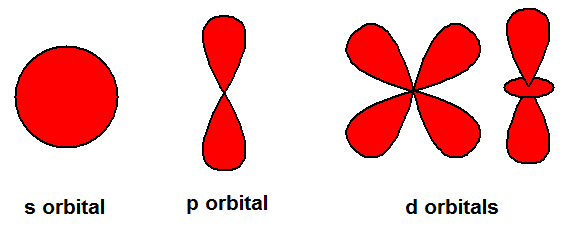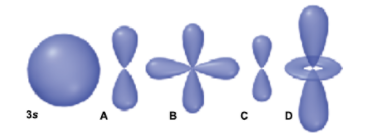# Problem: Use the relative size of the 3s orbital represented below to answer the following question.Which orbital has the highest energy?a. Ab. Bc. Cd. D

###### FREE Expert Solution
99% (353 ratings)
###### FREE Expert Solution

We’re being asked which of the given orbitals has the highest energy. Let’s first determine the shapes of the s, p, and d orbitals.Looking at each option below:

99% (353 ratings)###### Problem Details

Use the relative size of the 3s orbital represented below to answer the following question.

Which orbital has the highest energy?

a. A

b. B

c. C

d. DWhat scientific concept do you need to know in order to solve this problem?

Our tutors have indicated that to solve this problem you will need to apply the Orbital Shapes concept. You can view video lessons to learn Orbital Shapes. Or if you need more Orbital Shapes practice, you can also practice Orbital Shapes practice problems.

What is the difficulty of this problem?

Our tutors rated the difficulty ofUse the relative size of the 3s orbital represented below to...as medium difficulty.

How long does this problem take to solve?

Our expert Chemistry tutor, Sabrina took 2 minutes and 26 seconds to solve this problem. You can follow their steps in the video explanation above.

What professor is this problem relevant for?

Based on our data, we think this problem is relevant for Professor Wall's class at VT.